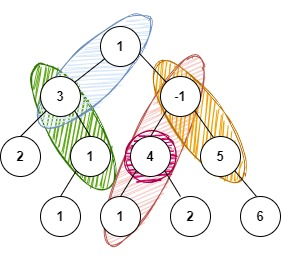# K - Sum Path In A Binary Tree

Posted: 29 Nov, 2020
Difficulty: Moderate

## PROBLEM STATEMENT

#### Note:

``````1. The path does not need to start or end at the root or a leaf, but it must go downwards (traveling only from parent nodes to child nodes).

2. Output the paths in the order in which they exist in the tree from left to right. Example: In the below example, path {1,3} is followed by {3,1} and so on.
``````

#### Example:

``````For K = 4 and tree given below:
````````````The possible paths are:
1 3
3 1
-1 4 1
4
-1 5

The sum of values of nodes of each of the above-mentioned paths gives a sum of 4.
``````
##### Input format
``````The first line of input contains an integer ‘T’ denoting the number of test cases.
The next ‘T’ lines represent the ‘T’ test cases.

The first line of input contains the elements of the tree in the level order form separated by a single space.

If any node does not have a left or right child, take -1 in its place. Refer to the example below.

The second line of each test case contains an integer ‘K’ denoting the sum.
``````
##### Example:
``````Elements are in the level order form. The input consists of values of nodes separated by a single space in a single line. In case a node is null, we take -1 in its place.

For example, the input for the tree depicted in the below image would be :
```````````` 1
2 3
4 -1 5 6
-1 7 -1 -1 -1 -1
-1 -1
``````

Explanation : Level 1 : The root node of the tree is 1

``````Level 2 :
Left child of 1 = 2
Right child of 1 = 3

Level 3 :
Left child of 2 = 4
Right child of 2 = null (-1)
Left child of 3 = 5
Right child of 3 = 6

Level 4 :
Left child of 4 = null (-1)
Right child of 4 = 7
Left child of 5 = null (-1)
Right child of 5 = null (-1)
Left child of 6 = null (-1)
Right child of 6 = null (-1)

Level 5 :
Left child of 7 = null (-1)
Right child of 7 = null (-1)

The first not-null node (of the previous level) is treated as the parent of the first two nodes of the current level. The second not-null node (of the previous level) is treated as the parent node for the next two nodes of the current level and so on.

The input ends when all nodes at the last level are null (-1).
``````
##### Note :
``````The above format was just to provide clarity on how the input is formed for a given tree.

The sequence will be put together in a single line separated by a single space. Hence, for the above-depicted tree, the input will be given as:

1 2 3 4 -1 5 6 -1 7 -1 -1 -1 -1 -1 -1
``````
##### Output format:
``````The output of each test case prints all the paths of the binary tree with the sum of nodes in the path as ‘K' in the order in which they exist in the tree from left to right.

The output of each test case is printed in a separate line.
``````
##### Note
``````You are not required to print anything; it has already been taken care of. Just implement the function and return the answer.
``````
##### Constraints:
``````1 <= T <= 50
1 <= N <= 10^2
-10^9 <= K <= 10^9
-10^7 <= DATA <= 10^7

Where ‘N’ is the number of nodes in the tree, ‘K’ denotes the sum, and 'DATA' denotes data contained in the node of a binary tree.

Time Limit: 1 sec
``````Approach 1

The idea is to generate all possible paths and check whether any path sum is equal to ‘K’ or not.

1. If the ‘ROOT’ is NULL, return.
2. Else, add the current node value to a vector.
3. Recur for the left subtree.
4. Recur for the right subtree.
5. Traverse the vector from the back. The last element is taken as the node at which our current path terminates. Keep adding the elements of the vector and if the sum becomes equal to ‘K’, print the path.
6. To backtrack remove the last element from the vector.